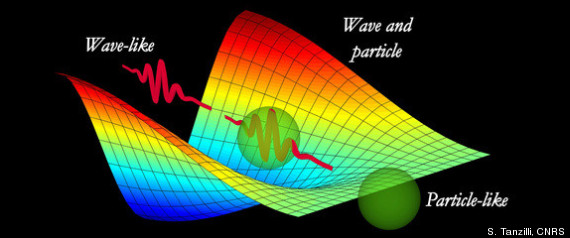# Messing with an electronAn electron, practically at rest is initially accelerated through a potential difference $100 \text{ V}$. It then has a de Broglie wavelength of $x_1$.

It then gets retarded through $19 \text{ V}$ and has a wavelength of $x_2$.

A final retardation through $32 \text{ V}$ changes the wavelength to $x_3$.

What is $\displaystyle\frac{x_3-x_2}{x_1}$ as a percentage?

×